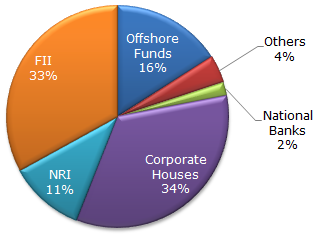# Data Interpretation - Pie Charts - Discussion

The following pie chart shows the amount of subscriptions generated for India Bonds from different categories of investors.

Subscriptions Generated for India Bonds1.

In the corporate sector, approximately how many degrees should be there in the central angle ?

 [A]. 120 [B]. 121 [C]. 122 [D]. 123

Explanation:

34 x 3.6 = 122.4 (since 1% = 3.6 degrees)

 Naveen said: (Oct 22, 2012) Can anyone please elaborate? why do we need to take 34 above?

 Krishnaveni said: (Oct 21, 2013) Corporate houses is 34%. Central angle = 360. So, there fore 34/100*360 = 34*3.6 = 122.4.

 Rajendra said: (Dec 31, 2016) In case otherwise, 34% of 360 degree will be 122.4 degree. i.e. 360*34% = 122.4 degree.

 Kavita Pavar said: (Apr 5, 2019) If the offshore fund is 16% is the central angle then it could be 16*3.6.